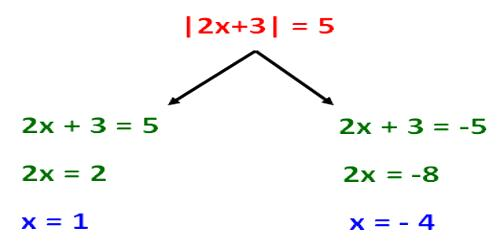Absolute Value of an Integer

The absolute value of an integer is the numerical value without regard to whether the sign is negative or positive. On a number line it is the distance between the number and zero.

Absolute value describes the distance of a number on the number line from 0 without considering which direction from zero the number lies. The absolute value of a number is never negative. The absolute value of 5 is 5.

The absolute value of -15 is 15. The absolute value of +15 is 15

The symbol for absolute value is to enclose the number between vertical bars such as |-20| = 20 and read “The absolute value of -20 equals 20”.Absolute value describes the distance of a number on the number line from 0 without considering which direction from zero the number lies. The absolute value of a number is never negative.

Symbol used in absolute value

The symbol used to denote the absolute value is, two vertical lines (| |), one on either side of an integer.

Therefore, if ‘a’ represents an integer, its absolute value is represented by |a| and is always non-negative

Note:

(i) |a| = a; when ‘a’ is positive or zero.

(ii) |a| = -a; when ‘a’ is negative.

Examples on absolute value of an integer:

(i) Absolute value of – 7 is written as |- 7| = 7 [here mod of – 7 = 7]

(ii) Absolute value of + 2 is written as |+ 2| = 2 [here mod of + 2 = 2]

(iii) Absolute value of – 15 is written as |- 15| = 15 [here mod of – 15 = 15]

(iv) Absolute value of + 17 is written as |+ 17| = 17 [here mod of + 17 = 17]

Sample Problem

What’s the value of -|-5|?

We’ve got two negative signs and an absolute value sign. Let’s work our way from the inside out. Remember, anything inside those absolute value bars is positive.

-|-5| = -(5) = -5

Information Source: